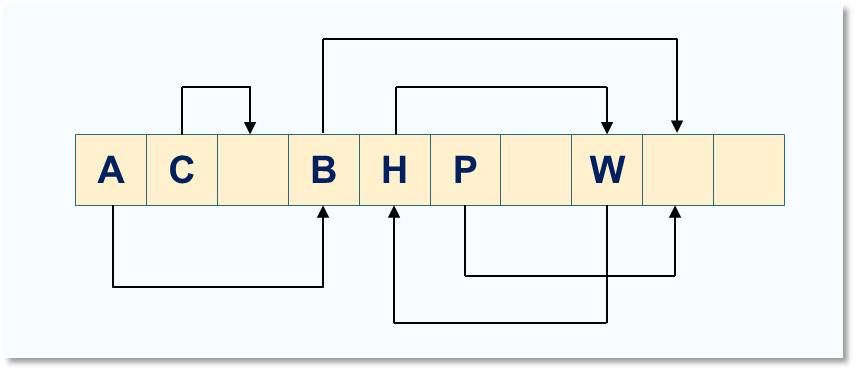# How Cuckoo Hashing Works

The Cuckoo hashing algorithm allows for lookup of items at constant time, O(1). We would cover the following in this article:1. How the Cuckoo Hashing Works

In cuckoo hashing, we maintain two hash position (and hash functions) h1 and h2.  So if we need to store a key x, we would compute one of two postions h1(x) and h2(x).

This means that to retrieve an item, we simply need to lookup just two positions in the table. This is shown in Table 1.0.Figure 1.0: Cuckoo hash table. The arrow directions shown the alternative location of each of the items in the table. If a new item is to be inserted into position occupied by A, then A is ejected and moved to its second alternative position. Insertion in position of H would lead to a cycle and would require to rehash the entire table.

To insert an Item, we first try to insert the item into position h1(x). If the position is empty, we insert the item. But if it happens that the position if occupied, we throw out the existing key in that location and insert x. (This is the behaviour of the Cuckoo). Assuming the ejected key is y, then y is place in the alternative position which is h2(y). If also this position is occupied by a key z, the y repeats the same treatment: throws out z to make room. This process continues until a given threshold is reached.

In situation like this, if cycles are encountered, then we choose new has functions h1() and h2 and rehash or rebuild the entire table.

2. The Cuckoo Hashing Algorithm

The cuckoo hashing algorithm is given in Listing 1.0. The variable pos is used to maintain the current position where we are currently trying to insert an item.

```Procedure insert (x)
if(T[h1(x) = x or T[h2(x) = x
then return
else
pos gets h1(x)
Loop n times {
if T[pos] = NULL
then T[pos] gets x;
return
else
swap x and T[pos]
if pos = h1(x) then post gets h2(x) else post gets h1(x)
rehash(); insert(x)
End
```

Listing 1.0: Cuckoo Hashing Algorithm

3. The Cuckoo Graph

A cuckoo graph is formed from the movement of the keys between alternative positions in the hash table. This can be created from Figure 1.0. In the figure, the direction of the arrow on each of the edges indicate that the element can move along the edge to its alternative position

When inserting an item x, the insertion algorithm will only visit positions to which there is a path in the cuckoo graph from either of the two positions h1(x) and h2(x). In the event of a loop, then we go ahead to rehash.

Analysis of the cuckoo graph shows that insertion takes constant time in the absence  of a rehashing.

4. Cycles in Cuckoo Graph

Assuming we want to insert a key Z. We would examine the  two possible position h1(Z) and h2(Z) which are called buckets. Let’s also assume that h1(Z) hashes to W. Then then:

• W is moved to position of H
• H is moved to position of of H and this becomes a cycle which would require rehashing the table

If however h1(Z) hashes to the position of W and W moves to the position of A. Then a can move to position of B and B moves to an empty slot. In this case, there is not cycles.

5. Demo on the Cuckoo Hashing

##### Check these Data Structure and Algorithms Tutorials#### kindsonthegenius

Kindson Munonye is currently completing his doctoral program in Software Engineering in Budapest University of Technology and Economics

View all posts by kindsonthegenius →

## 2 thoughts on “How Cuckoo Hashing Works”

1.ReyesBig says:

Hello. I have checked your kindsonthegenius.com and i see you’ve got
some duplicate content so probably it is the reason that you don’t
rank hi in google. But you can fix this issue fast.

There is a tool that creates articles like human, just search in google:
miftolo’s tools